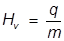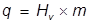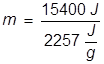# Heat of Vaporization Formula

Heat of Vaporization Formula

When a liquid substance turns into a gas it is known as vaporization. The vaporization process requires an increase in energy to allow the liquid particles to overcome intermolecular attractions and vaporize. The amount of energy required is called the heat of vaporization. The heat of vaporization is different for all substances, but is a constant for each individual substance.Hv = heat of vaporization

q = heat

m = mass

Heat of Vaporization Formula Questions:

1. If the heat of vaporization for water is 2257, how much heat energy must be applied to vaporize 135 grams of water?

The heat of vaporization equation is rearranged to solve for q.2. What mass of water can be vaporized at 100°C if 15400 J of heat energy is applied?

The heat of vaporization for water is applied here and the equation is rearranged to solve for mass.